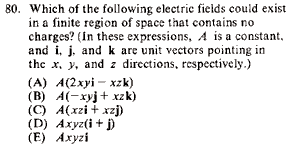GR 8677927796770177 | # Login | Register

GR8677 #80
Problem
 GREPhysics.NET Official Solution Alternate SolutionsElectromagnetism}Gauss Law

Recall the differential form of Gauss' Law . For fields that contain no charges, the equation becomes . Find the choice with 0 divergence.

(A)

(B) ... so this is it!

(C)

(D)

(E)Alternate Solutions
 casseverhart132019-08-23 12:20:38 I really appreciate this problem....thanks.... tree service OrlandoReply to this commentnakib2010-04-02 09:01:27 Don't go for calculating all the answer choices for . Eliminate C, D ~> Each term has a non-zero contribution to the div. but both terms are +ve, they can't possible cancel each other out. Eliminate E ~> Just one term that has a non-zero contribution to the div. Eliminate A ~> Each term has a non-zero contribution to the div. but one term has a factor of multiplied to it. (B) is the correct choice.Reply to this commentsenatez2006-10-16 20:25:28 The electric field must also be a gradient of a function (the potential). Mixed partials of the potential function must be equal.Reply to this commentcasseverhart13
2019-08-23 12:20:38
I really appreciate this problem....thanks.... tree service Orlandonakib
2010-04-02 09:01:27
Don't go for calculating all the answer choices for .

Eliminate C, D ~> Each term has a non-zero contribution to the div. but both terms are +ve, they can't possible cancel each other out.

Eliminate E ~> Just one term that has a non-zero contribution to the div.

Eliminate A ~> Each term has a non-zero contribution to the div. but one term has a factor of multiplied to it.

(B) is the correct choice.
 neon372010-11-04 04:40:24 Its not too hard if you think about how to do it fast before hand. It might take longer to think about some other logic. Divergence is a dot product, so for terms you take . That shouldnt be too difficult. In this case it was just the second one, so it took me about 7 seconds maximum after reading the question.senatez
2006-10-16 20:25:28
The electric field must also be a gradient of a function (the potential). Mixed partials of the potential function must be equal.
 student20082008-10-12 12:08:08 Not necessarily. Since , you're right only in the electrostatic case, which takes place here. And in general we can't get rid of the by a gauge transformation, since the condition implies scalar ones. Although, since there're only expressions for the electric field, we can infer that the problem electrostatic.
 Prologue2009-11-06 21:03:53 You can't infer that it is electrostatic, the curl is nonzero.LaTeX syntax supported through dollar sign wrappers $, ex.,$\alpha^2_0$produces . type this... to get...$\int_0^\infty\partial\Rightarrow\ddot{x},\dot{x}\sqrt{z}\langle my \rangle\left( abacadabra \right)_{me}\vec{E}\frac{a}{b}\$